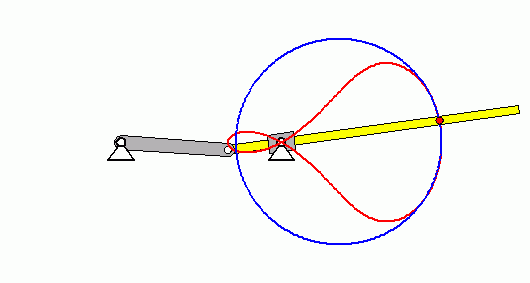# What is the curvature radius

### Circles of curvature of plane curves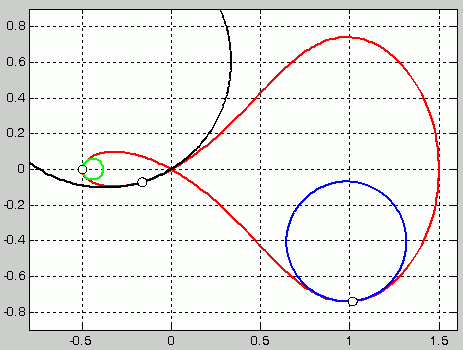Circles of curvature on a trajectory

The circle of curvature hugs a curve in the vicinity of a point of contact. The figure on the right shows the (blue, black or green) circles of curvature for three points of the curve (drawn in red) (the curve is the trajectory of a point on an oscillating crank loop, see the animation below). As a rule, for each point on a curve there is a different circle of curvature with its own center of curvature and its own radius of curvature.

What do you need that for?

In technical mechanics, the radius of curvature plays an important role in various areas, for example:

• In the case of a bending beam, the bending moment is inversely proportional to the radius of curvature of the deformed beam. On this basis, the non-linear differential equation of the bending line is derived in the chapter "Deformations due to bending moments".
• The normal acceleration when moving on a curved path is calculated according to the formula an= v2/ ρ calculated (with the path velocity v and the radius of curvature of the path curve ρ). This is usually the most important (because it is by far the largest) component of the overall acceleration.

### Definitions

The formulation of "optimal snuggling" used above can be put into mathematically usable definitions in different ways. The first definition is based on the definition of the tangent on a curve: Two points clearly define a straight line, which is called a secant if the two points lie on a curve. If these two points come closer and closer together until they finally coincide at the border crossing, then the secant becomes the tangent to the curve at this point. The following is defined in the same way:

Definition 1: A circle can be clearly drawn through three points of a (plane) curve that are not on a straight line. When the distances between these points become smaller and smaller and finally approach zero, the circle defined by this limit crossing becomes the Circle of curvature called the curve at this point.

The following definition is completely equivalent to this:

Definition 2: A circle that has this point in common with a point on a curve y = f (x), the tangent at this point (first derivative y ') and the second derivative y' ' Circle of curvature called the curve at this point.

Definition 2 is used below to derive the required formulas.

### Formulas for the radius of curvature

A function in parametric representation is given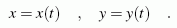This is not a restriction of generality, because every function y = f (x) can also be represented as x = t & nbsp, & nbsp y = f (t). A circle is searched for (the parameter representation is also used for this)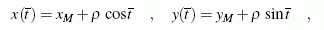which also corresponds to this function in a point given by the coordinates x and y in the two derivatives y 'and y' '. These derivatives are formed for the circle: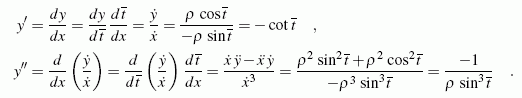Out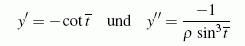t can be eliminated, and one obtains a formula for ρ: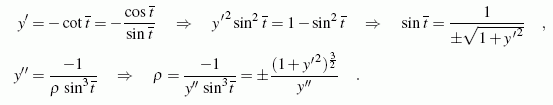In a corresponding way you get from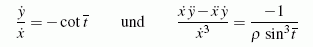a formula for the parametric representation of the function. Because a radius must of course have a positive value, the absolute value is taken for the second derivative, which can be positive or negative, and the following result ...

#### Formulas for the radius of curvature: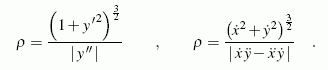The function for which a radius of curvature is sought can be given as y & nbsp = & nbspf (x) (then the formula on the left is responsible) or in parametric representation as x (t) & nbsp, & nbspy (t) (then the formula on the right is used) . After creating the derivatives, the coordinates of the point must be inserted for which the radius of curvature is to be calculated. The reciprocal of the radius of curvature is called the curvature of the curve at the corresponding point.

### Coordinates of the center of curvature

The function of the circle of curvature, written down in general form above, is converted according to the coordinates of the center point: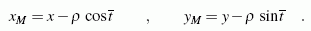In order to avoid the sign problem with the root, we refer to the intermediate results achieved above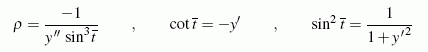are used, which can be used to obtain the following formulas, for example: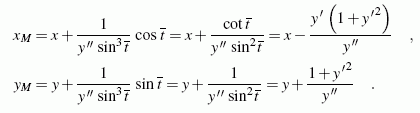For the function given in the parametric representation, analog formulas are calculated in a corresponding way;

#### Coordinates of the center of curvature: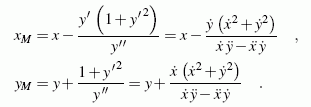Here, too, after the derivatives have been created, the coordinates of the point must be inserted on the right-hand side for which the center point coordinates of the circle of curvature are to be calculated.

#### Evolute and involute

The geometric location of all centers of curvature of a curve is called the evolute, the curve itself is the involute of this evolute. These curves play an important role in the gear geometry.

### example 1

Calculate for the function y & nbsp = & nbspax2 generally the radius of curvature ρ (x) and the coordinates of the centers of curvature xM.(x) and yM.(x). For a = 0.5, the radii of curvature that belong to points (0; 0) or (1; 0.5) and the parametric representation of the evolute must be specified.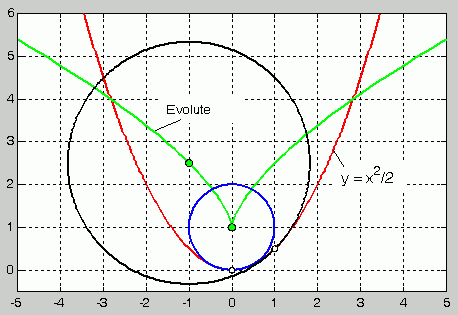Function (drawn in red) with two circles of curvature and Evolute

The function and its first two derivatives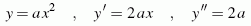are used in the formulas given above: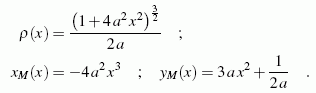For a = 0.5 the two special radii of curvature are calculated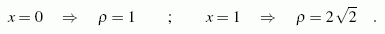The calculated formulas for the coordinates of the centers of curvature xM.(x) and yM.(x) can be viewed as a parametric representation (parameter is x) of the Evolute. For a = 0.5 one obtains with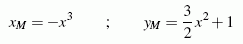the parametric representation of the Evolute. In the graphic above, the function y = x2/ 2 red, the two special circles of curvature blue and black and the evolute green.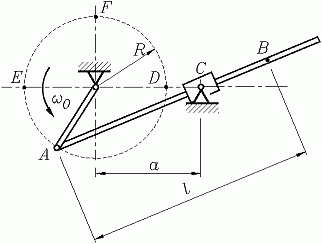### Example 2

The mechanism sketched opposite is a so-called "swinging crank loop". The crank rotates with the constant angular velocity ω0.

Given: R; ω0 ; a / R = 1.5; l / R = 2.

1. For a complete revolution of the crank, the radius of curvature ρ (φ), the path speed of the point Bv (φ), its tangential acceleration are at(φ) and normal acceleration an(φ) graphically (φ = ω0t).
2. The trajectory of point B should be shown and it should be made clear that the radius of curvature changes constantly with such a curve.

The determination of the trajectory is required as a question of a task from the chapter "Kinematics of the point". The result can be found on the Internet in the section "Solutions to the exercises". The curve can only be given meaningfully in the parametric representation. With a Cartesian coordinate system with the origin at point C, if point A of the crank is at point D at time t = 0: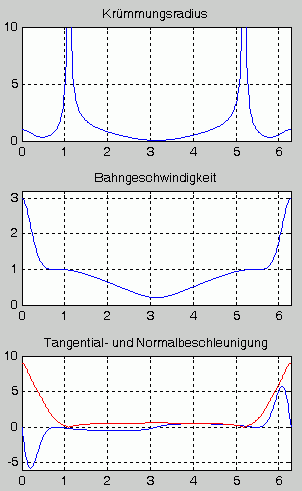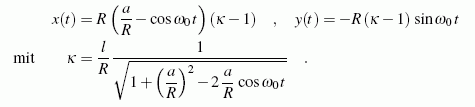The formation of the first two derivatives of these functions, which are required for writing down the radius of curvature and the center of curvature, is quite laborious (but can still be mastered "by hand"). It is well advised to get help from the computer.

The (tangentially directed) path speed is calculated from the speed components, and the tangential acceleration is the derivation of the path speed in terms of time: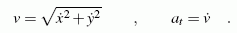For the normal acceleration (perpendicular to the tangent to the trajectory)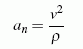the radius of curvature is also required, which is calculated using the formulas given above. The graphic representation of the functions can be seen on the right. It can be seen that the normal acceleration (shown in red) takes on greater values ​​than the tangential acceleration. Their maximum value is not in the area of ​​particularly small radii of curvature, because the square of the speed in the numerator of the fraction has a greater influence. It is typical that the tangential acceleration can assume positive and negative values, the normal acceleration, on the other hand, is always given as positive because it is always directed towards the center of curvature.

In the animation below you can see the red trajectory of point B and the constantly changing (blue drawn) circles of curvature: